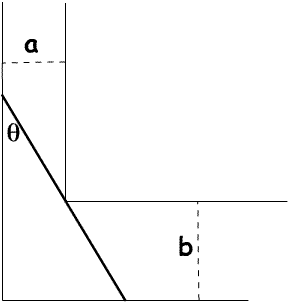#### You may also like### Generally Geometric

Generalise the sum of a GP by using derivatives to make the coefficients into powers of the natural numbers.### Exponential Trend

Find all the turning points of y=x^{1/x} for x>0 and decide whether each is a maximum or minimum. Give a sketch of the graph.### Slide

This function involves absolute values. To find the slope on the slide use different equations to define the function in different parts of its domain.

# Bend

##### Age 16 to 18 Challenge Level:This solution comes from Andrei Lazanu ,Tudor Vianu National College, Bucharest, Romania.

I calculate the length of the stick in terms of $a$, $b$ and $\theta$. From the figure I observe that the length of the stick could be seen as the sum of two hypotenuses of two right-angled triangles. Its length is: $$l(\theta)= {a\over \sin \theta} + {b\over \cos \theta}.$$ Now, I have to calculate the minimum of this expression, in order to make the stick pass through the corner. For this, I calculate the derivative of $l(\theta)$ and equate it to $0$. I must say from the beginning that derivatives are not so familiar to me. For a minimum value of the length: $${\rm{d}l\over \rm{d}\theta} = {-a\cos \theta \over \sin^2 \theta} + {b\sin \theta \over \cos^2 \theta}=0.$$ So for a minimum value $a\cos^3 \theta = b\sin^3 \theta$ and $$\tan \theta = \left({a\over b}\right)^{1/3}.$$ Now, I have to calculate $\sin\theta$ and $\cos\theta$ as functions of $\tan\theta$. I know that: $$\cos x = {1\over \sqrt{1+\tan^2x}}$$ and$$\sin x = {\tan x \over \sqrt{1+\tan^2x}}$$ In the case of the problem, I have: $${1\over \cos \theta }= \sqrt{1+\left({a\over b}\right)^{2/3}}$$ and $${1\over \sin \theta } = \left({b\over a}\right)^{1/3}\sqrt{1+\left({a\over b}\right)^{2/3}}$$ So the minimum length is \eqalign{ {a\over \sin \theta} + {b\over \cos \theta} &=\left(a^{2/3}b^{1/3} + b\right)\sqrt{{a^{2/3}+b^{2/3}\over b^{2/3}}} \cr &= \left(a^{2/3} + b^{2/3}\right)^{3/2}}. The result is symmetric in $a$ and $b$.

If $a=65 \text{ cm}$ and $b=75 \text{ cm}$ then $65^{2/3}+ 75^{2/3}=16.16623563 + 17.78446652=33.95070215$ and $33.951^{3/2}=197.8213407$ so an object of about $197 \text{ cm}$ could be manoeuvred around the bend but it is not possible to manoeuvre a $200 \text {cm}$ object around this bend.